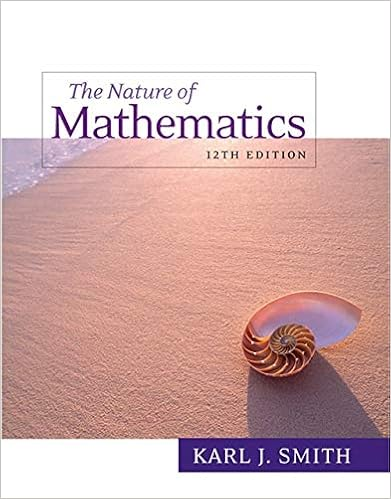# REVIEW FOR EXAM CALC II.docx - SECTION 6.1 Deceleration A...

• 13

This preview shows page 1 - 5 out of 13 pages.

##### We have textbook solutions for you!
The document you are viewing contains questions related to this textbook.The document you are viewing contains questions related to this textbook.
Chapter 10 / Exercise 28
Nature of Mathematics
SmithExpert Verified
SECTION 6.1DecelerationA car slows down with an acceleration ofa(t) = -15 ft/s2. Assume that v(0) = 60 ft/s, s(0) = 0, and tis measured in seconds.a. Determine and graph the position function, for t >= 0.b. How far does the car travel in the time it takes to come to rest?SECTION 6.2Sketch the region and find its area9. The region bounded by y = 2(x + 1), y = 3(x + 1), and x = 410. The region bounded by y = cos x and y = sin x between x = pi/4 and x = 5pi/4
##### We have textbook solutions for you!
The document you are viewing contains questions related to this textbook.The document you are viewing contains questions related to this textbook.
Chapter 10 / Exercise 28
Nature of Mathematics
SmithExpert Verified
SECTION 6.327–34. Washer method Let R be the region bounded by the followingcurves. Use the washer method to find the volume of the solid generatedwhen R is revolved about the x-axis.31. y = x + 3, y = x2+ 132. y = sqrt (sin x), y = 1, x = 033. y = sin x, y = sqrt (sin x), for 0 <=x <= pi/234. y = | x | , y = 2 – x2
SECTION 6.45–14. Shell methodLet R be the region bounded by the following
•••